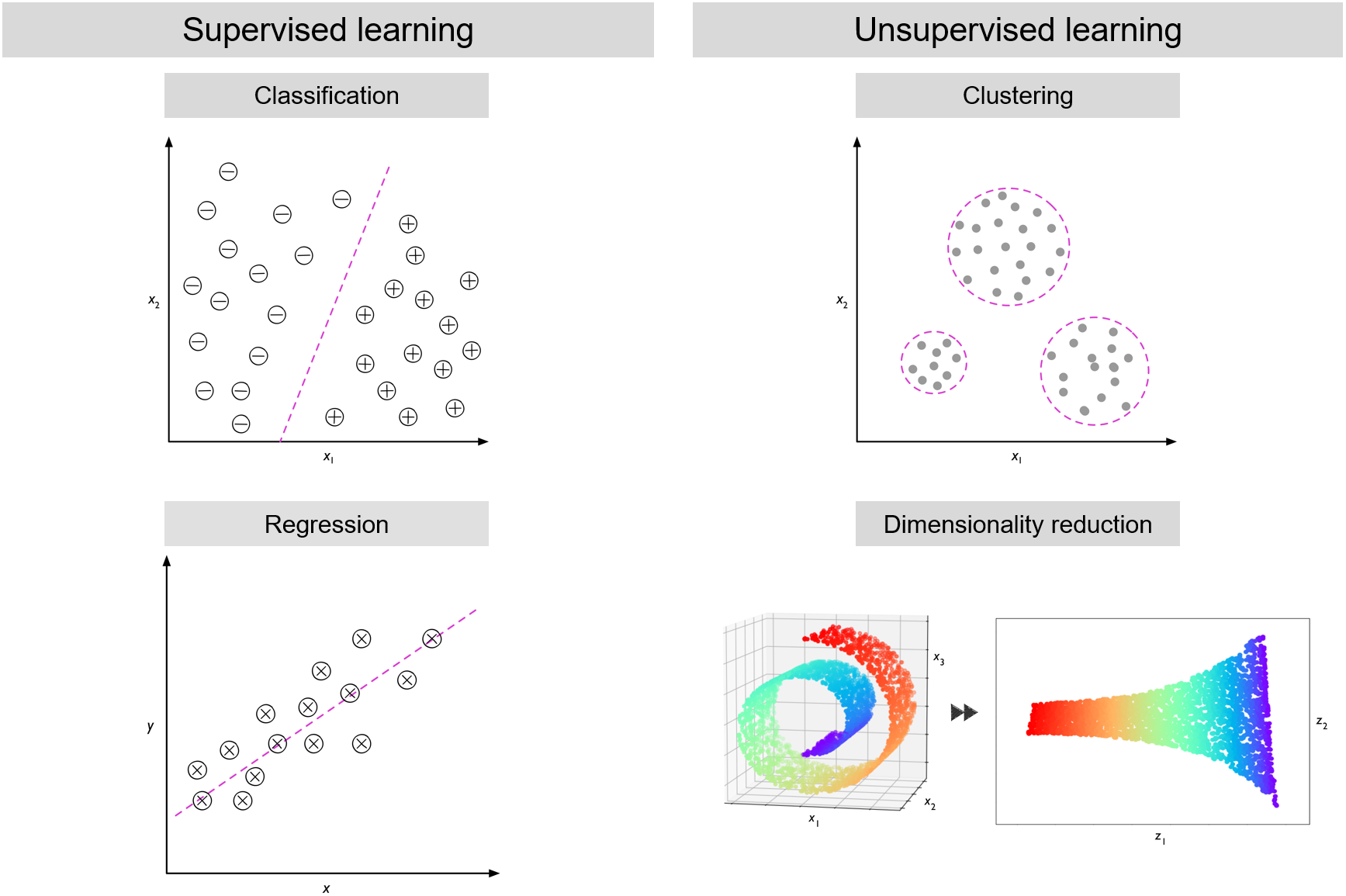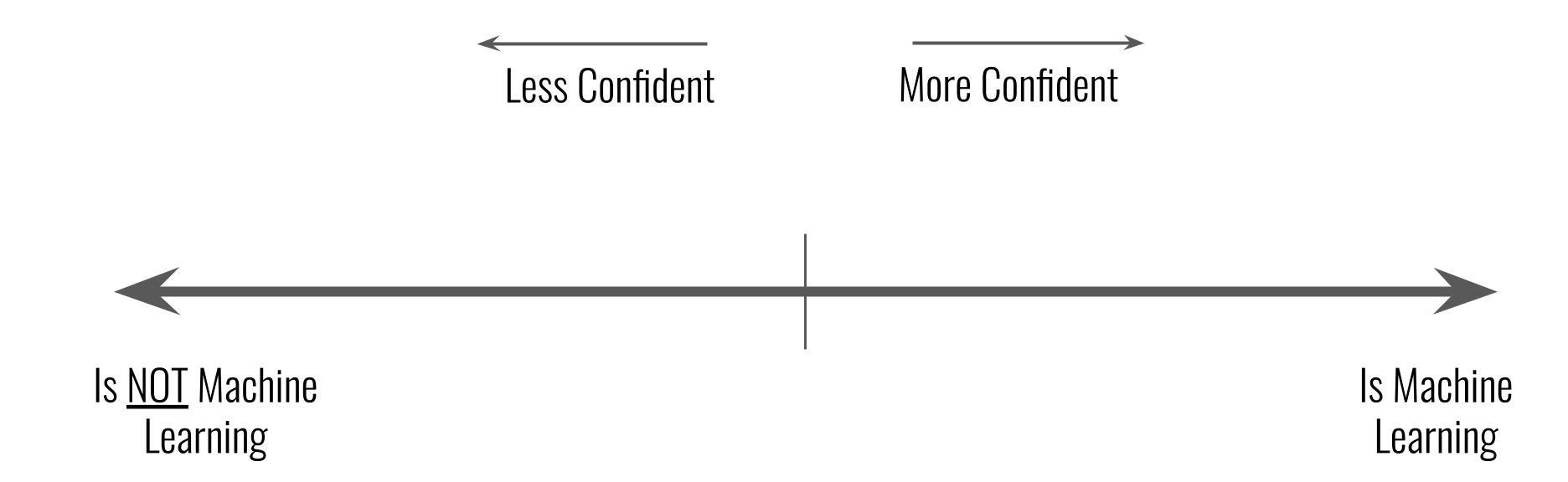This lesson is being piloted (Beta version)

# Introduction

## Overview

Duration: 35 min
Questions
• What is machine learning?

Objectives
• Define and give examples of machine learning

• Identify problems in computational biology suitable for machine learning

### What is this workshop about?

The learning objectives of this workshop are:

1. Identity and characterize machine learning and a machine learning workflow.

2. Evaluate whether a particular problem is easy or hard for machine learning to solve.

3. Assess a typical machine learning methodology presented in an academic paper.

4. Gain confidence in and appreciation for machine learning in biology.

We will also be learning about some specific machine learning models: decision trees, random forests, logistic regression, and artificial neural networks.

## Definition

Machine learning is a set of methods that can automatically detect patterns in data and then use those patterns to make predictions on future data or perform other kinds of decision making under uncertainty.

A machine learning algorithm gets better at its task when it is shown examples as it tries to define general patterns from the examples.

One of the most popular textbooks on machine learning, Machine Learning by Tom Mitchell, defines machine learning as, “the study of computer algorithms that improve automatically through experience.”

## Definition

Algorithm - is a relationship between input and output. It is a set of steps that takes an input and produces an output.

Machine learning can be broadly split into two categories, supervised machine learning and and unsupervised learning. Machine learning is considered supervised when there is a specific answer the model is trying to predict, questions like what is the price of a house? Is this mushroom edible? What disease does this patient have? Some examples of supervised machine learning are classification and regression. These will be further defined in the next lesson.

Unsupervised machine learning has no particular target for its learning, it is instead trying to answer general questions about patterns. This can include questions like how do these cells group together? In what way are these samples most different from each other? An example of unsupervised machine learning is clustering. Unsupervised learning will not be covered in this workshop. For some external resources, check out the references.

## Definitions

Supervised learning - training a model from the labeled input data.

Unsupervised learning - training a model from the unlabeled input data to find patterns in the data.Image adapted from Python Machine Learning, 2rd Ed. by Sebastian Raschka and Vahid Mirjalili

## Definition

Clustering - grouping related samples in the data set.

Classification - predicting a category for the samples in the data set.

Regression - predicting a continuous number for the samples in the data set.

Classifier - a specific model or algorithm that performs classification.

Model - mathematical representation that generates predictions based on the input data.

## Scenarios

Consider whether the following 3 scenarios should or should not be considered machine learning.

1. You are trying to understand how temperature affects the speed of embryo development in mice. After running an experiment where you record developmental milestones in mice at various temperatures, you run a linear regression on the results to see what the overall trend is. You use the regression results to predict how long certain developmental milestones will take at temperatures you’ve not tested.

2. You want to create a guide for which statistical test should be used in biological experiments. You hand-write a decision tree based on your own knowledge of statistical tests. You create an electronic version of the decision tree which takes in features of an experiment and outputs a recommended statistical test.

3. You are annoyed when your phone rings out loud, and decide to try to teach it to only use silent mode. Whenever it rings, you throw the phone at the floor. Eventually, it stops ringing. “It has learned. This is machine learning,” you think to yourself.Confidence scale for machine learning scenarios.

### What is the difference between a machine learning algorithm and a traditional algorithm?

Traditional algorithm: Let’s say you are doing an experiment, and you need to mix up some solutions. You have all the ingredients, you have the “recipe” or the proportions, and you follow the recipe to get a solution.

Machine learning algorithm: You are given the ingredients and the final solution, but you don’t know the recipe. So, what you need to do it to find the “fitting” of the ingredients, that would result in your solution.

Think about the following questions whenever we encounter a situation involving machine learning.

### What does machine learning mean for biology?

Machine learning can scale, easily making predictions on a large number of items. It can be very slow and expensive for expert biologists to manually make the same decisions or manually construct a decision-making model. Training and executing a machine learning model can be faster and cheaper. Machine learning may also recognize complex patterns that are not obvious to experts.

Let’s look at some examples of how machine learning is being used in biology research.

A guide to machine learning for biologists is an excellent reference that covers many of the concepts discussed in this workshop.

## Key Points

• Machine learning algorithms recognize patterns from example data

• Supervised learning involves predicting labels from features